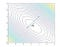# Working with contours using OpenCV

To be able to understand and work with an image we need to break it down into its constituent features. An image feature is a simple image pattern, based on which we can describe what we can see on the image.

The main of role of features in computer vision is to transform visual information into vector spaces this gives us the power to perform several mathematical operations on them.

## Let’s understand contours mathematically! 🥱🥱

A contour line of a function of 2 variables is locus of the points along which the function has constant value. Hence it is a plane section of a 3-dimensional graph of a function, say f(x,y).On any given circle any point will have the same functional value .

If we use the idea of contours in image processing, and apply contours on images then we will be tracing line of pixels with same value. In simple terms, the boundary! Yup, contours are boundaries of objects in an image.

## So how do we detect contours using OpenCV?

Follow the below mentioned steps to detect contours:

1. Let’s import all necessary libraries first.
`import cv2import numpy as npimport matplotlib.pyplot as plt`

2. Read the image using cv2 (default colour channel BGR) and make a gray scale copy of it. We will use thresholding which is only applied on grayscale images.

(For every pixel same threshold value is applied and if pixel value is smaller than the threshold than it is set to 0, otherwise it is set to maximum value.This is called thresholding.)

`img = cv2.imread("shape.jpg")imgray = cv2.cvtColor(img,cv2.COLOR_BGR2GRAY)thresh=cv2.adaptiveThreshold(imgray,255,cv2.ADAPTIVE_THRESH_GAUSSIAN_C, cv2.THRESH_BINARY,11,2)`

3. Now we will find the contours and draw them on the image using cv2.

`contours, hierarchy = cv2.findContours(thresh,cv2.RETR_TREE,cv2.CHAIN_APPROX_SIMPLE)ctr = np.zeros(img.shape, dtype=np.uint8)cv2.drawContours(ctr, contours, -1, (0,255,0), 3)img_with_contours = img.copy()cv2.drawContours(img_with_contours, contours, -1, (0,255,0), 3)`

4. You can see the contour plots for the images below by using matplotlib.

`f = plt.figure(figsize=(15,15))f.add_subplot(2, 2, 1).set_title('Original Image')plt.imshow(img[:,:,::-1])f.add_subplot(2, 2, 2).set_title('Thresholded Image')plt.imshow(thresh, cmap="gray")f.add_subplot(2, 2, 3).set_title('Contours standalone')plt.imshow(ctr[:,:,::-1])f.add_subplot(2, 2, 4).set_title('Original Imagewith contours')plt.imshow(img_with_contours[:,:,::-1])plt.show()`